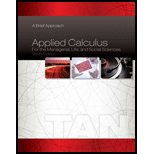Chapter 7.3, Problem 13E### Applied Calculus for the Manageria...

10th Edition
Soo T. Tan
ISBN: 9781285464640

#### Solutions

Chapter
Section### Applied Calculus for the Manageria...

10th Edition
Soo T. Tan
ISBN: 9781285464640
Textbook Problem

# In Exercises 1–14, use the Trapezoidal Rule and Simpson’s Rule to approximate the value of each definite integral. Compare your result with the exact value of the integral.13. ∫ 1 2 ln x d x ; n = 4

To determine

To approximate: The value of definite integral by using Trapezoidal Rule and Simpson’s Rule and compare the result with the exact value of the integral.

Explanation

Given:

The integral 12lnxdx and n=4 .

Formula used:

Trapezoidal Rule: abf(x)dxΔx2[f(x0)+2f(x1)+...+2f(xn1)+f(xn)]

Where Δx=ban and xn=a+nΔx .

Simpson’s Rule: abf(x)dxΔx3[f(x)+4f(x1)+2f(x2)+...+4f(xn1)+f(xn)]

Where Δx=ban and n is even.

Calculation:

Consider the integral 12lnxdx .

Here, a=1, b=2 and n=4 .

Substitute a=1, b=2 and n=4 in Δx=ban ,

Δx=214=14

The end point of the sub-intervals are x0=1, x1=54, x2=32, x3=74 and x4=2 .

The Trapezoidal Rule yields,

12lnxdx142[f(1)+2f(54)+2f(32)+2f(74)+f(2)]=18[ln(1)+2(ln(54))+2(ln(32))+2(ln(74))+ln(2)]=18[0+(2ln(5)2ln(4))+(2ln(3)2ln(2))+(2ln(7)2ln(4))+ln(2)]=18[0+0.446287+0.810930+1.119232+0.693147]

Simplify further,

12lnxdx=3

### Still sussing out bartleby?

Check out a sample textbook solution.

See a sample solution

#### The Solution to Your Study Problems

Bartleby provides explanations to thousands of textbook problems written by our experts, many with advanced degrees!

Get Started

## Additional Math Solutions

#### Find more solutions based on key concepts